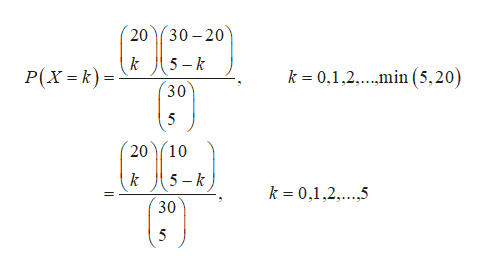# A fishpond contains 20 orange fish and 10 green fish. A sample of five fish is taken (withoutreplacement) from the pond. Let X denote the number of orange fish in the sample.(i) State the type of distribution of X, and write down its mass function.(ii) Hence find P(X = 2).(iii) If the sample contains at least four orange fish, how likely is it to contain only orangefish?

Question

A fishpond contains 20 orange fish and 10 green fish. A sample of five fish is taken (without
replacement) from the pond. Let X denote the number of orange fish in the sample.
(i) State the type of distribution of X, and write down its mass function.
(ii) Hence find P(X = 2).
(iii) If the sample contains at least four orange fish, how likely is it to contain only orange
fish?

check_circleExpert Solution
Step 1

(i)

Let us define the random variable X as the number of orange fish in the sample.

From the given information, there are 20 orange fishes and 10 green fish. And a sample of 5 fishes are selected without replacement. Here, the sample is selected without replacement and the population is finite. Hence, the suitable distribution is hyper geometric distribution.

The probability mass function for hyper geometric distribution is,

Step 2

Here, N=30(=20+10), M=20 and n=5.

The probability mass function for random variable X is,help_outlineImage Transcriptionclose30 20 k Р(х - к) - 5 k k= 0,1,2..min ( 5,20) 30 5 В") 20 10 5- k k k 0,1.2.5 30 20 fullscreen
Step 3

(ii)

The value of P(X=2) is 0.1599 and it is ...

### Want to see the full answer?

See Solution

#### Want to see this answer and more?

Solutions are written by subject experts who are available 24/7. Questions are typically answered within 1 hour*

See Solution
*Response times may vary by subject and question
Tagged in

### Basic Probability